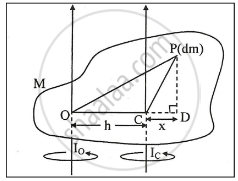Share

# State the Theorem of Parallel Axes About Moment of Inertia. - Physics

#### Question

State the theorem of parallel axes about moment of inertia.

#### Solution

Defination of moment of inertia :

A measure of the resistance of a body to angular acceleration about a given axis that is equal to the sum of the products of each element of mass in the body and the square of the element's distance from the axis.

Theorem of parallel axes:-

The moment of inertia of a body about any axis is equal to the sums of its moment of inertia about a parallel axis passing through its centre of mass and the product of its mass and the square of the perpendicular distance between the two parallel axes.

Mathematically , Io = Ic + Mh2

where Io = M. I of the body about any axis passing through centre O.

Ic = M. I of the body about parallel axis passing through centre of mass.

h = distance between two parallel axes.

Proof :

i) Consider a rigid body of mass M rotating about an axis passing through a point O as shown in the following
figure.
Let C be the centre of mass of the body, situated at distance h from the axis of rotation.ii) Consider a small element of mass dm of the body, situated at a point P.

iii) Join PO and PC and draw PD perpendicular to OC when produced.

iv) M.I of the element dm about the axis through O is (OP)2 dm

∴ M.I of the body about the axis thorugh O is given by

Io = ∫ (OP)2 dm         ......(1)

v) M.I of the element dm about the axis through c is CP2
dm

∴  M.I of the body about the axis through C

Ic = ∫ (CP)2 dm      .....(2)

vi) From the figure,

OP2 = OD2 +  PD2

= (OC + CD)2 + PD2

= OC2 + 2OC . CD + CD2 + PD2

∵ CP2 = CD2 + PD2

∴ OP2 = OC2 + 2 OC . CD + CP2    .....(3)

vii) From equation (1)

Io = ∫ (OP)2 dm

From equation (3)

"I"_o = int ("OC"^2 + 2 "OC" . "CD" + "CP"^2) "dm"

therefore "I"_o = int ("h"^2 + 2"hx" + "CP"^2) "dm"

= int  "h"^2 "dm" + int 2 "h.x"  "dm" + int  "CP"^2  "dm"

= "h"^2 int "dm" + 2"h" int  "x"  "dm" + int  "CP"^2 "dm"

"I"_o = "h"^2 int  "dm" + 2"h" int  "x"  "dm"

[From equation (2)]

therefore "I"_"o" = "I"_"c" + "h"^2 int  "dm" + "2h"  int  "x dm"        .......(4)

viii) Since ∫ dm = M and ∫ x dm = 0 and

algebraic sum of the moments of the masses of its individual particles about the centre of mass is zero
for body in equilibrium.

∴ Equation (4) becomes

Io = Ic + Mh2

Hence proved.

Is there an error in this question or solution?